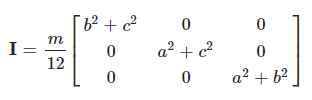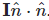# How do I calculate the MaxTorque property for a desired acceleration?

I’m trying to calculate the MaxTorque property of the “BodyAngularVelocity” object to make it rotate about a single-axis at a defined acceleration.

Here’s what I have so far:

``````local part = workspace.Part
local mass = part:GetMass()
local bVel = part.BodyAngularVelocity

function calculateMomentOfIntertia()
local size = part.Size

local x, y, z = size.X, size.Y, size.Z

return (mass * (z^2 + x^2)) / 12
end

function setAcceleration(targetAcceleration)
bVel.MaxTorque = calculateMomentOfIntertia() * targetAcceleration
end

setAcceleration(Vector3.new(0,1,0))
``````

It’s pretty much just a mess of physics equations I took from the internet and yields differing results depending on the scale of the part, so I’m clearly missing something.

What are you actually trying to do here?

Are you hacking the BodyAngularVelocity to be a kind of “BodyAngularForce” instead?

No, like I said in the topic; I’m trying to calculate the MaxTorque to produce a desired acceleration on a single axis.

The MaxTorque property indirectly defines the maximum acceleration used in reaching the AngularVelocity goal.

Right, just trying to clarify. If you really wanted to do this, you’d first at least need to set the BodyAngularVelocity’s `AngularVelocity` to be huge on the same axis as the torque, so that the velocity is constantly increasing. Something like

``````bVel.MaxTorque = -- I * accel
bVel.AngularVelocity = bVel.MaxTorque * 10000 -- always increase velocity
``````

But that seems like a weird and complicated thing to want to do, which is why I’m asking why you want to do it.

Also are you making the assumption that

1. the axes of rotation is always the Y axis, and
2. the part is always a cuboid?

If not “yes” to both, you’re going to have a worse timeThis physics stackexchange answer derives the equations for a cuboid about any axis: rotational dynamics - Determining the principal moment(s) of inertia and KE of a cuboid - Physics Stack ExchangeWhere a,b,c are the dimensions and m is the mass.

The inertia about axis n (as a unit vector) is then. You can then use that to calculate the torque like before.

(You should be able to use CFrames to do this matrix math)

You also may want to look at `BodyThrust`, which allows you to apply a torque to a part. You could put two of them on opposite sides to produce a constant torque about an axis. Might be easier that setting the MaxTorque of the body velocity, but you’ll still need those equations to calculate the force.

1 Like

Thank you for the detailed response!

To clarify; I’m trying to limit force applied in reaching a target AngularVelocity to a set acceleration; I’m not attempting to accelerate indefinitely.

I’ve applied your solution and I’m still getting varying accelerations depending on part scale.

``````function calculateIntertia()

local size = part.Size

local x, y, z = size.X, size.Y, size.Z

local a = z
local b = y
local c = x
return (mass/12) * Vector3.new(b*b + c*c, a*a + c*c, a*a + b*b)
end

function setAccel(accel)
bVel.MaxTorque = calculateIntertia() * accel
end
``````

I’m testing on a baseplate with zero gravity and a spinning cuboid part with different values for each size axis.
And I’m using the following script to benchmark the time it takes to reach the desired velocity:

``````local targetVel = Vector3.new(0,1,0)
local step = game:GetService('RunService').Stepped

wait(2) -- Wait a bit for roblox to start.

setAccel(Vector3.new(0,1,0))
bVel.AngularVelocity = targetVel

repeat
step:Wait()
until part.RotVelocity.Magnitude > 0 -- Wait for rotvelocity to start being applied.

local start = os.clock() -- Log the start timestamp.
repeat
step:Wait()
until part.RotVelocity.Magnitude >= targetVel.Magnitude -- Wait until the rotvelocity meets or exceeds the target rotvelocity.
print(os.clock() - start) -- Print the results.
``````

Obviously this sort of hacky benchmarking solution is going to yield results with some error, but it still should yield results in a relatively consistent way relative to the inputs.

Anyway, when I set the cuboid part size to (12, 1, 12) with a mass of 100.8; I get results around 1.16 seconds.

However, for example: when I scale up the Y axis of the part for a total size of (12, 587, 12) with a mass of 59,169.598; I get wildly different results at 2.083 seconds.

It’s important to note that there is 0 gravity in the workspace and the part is suspended above the ground (so there are no friction forces acting upon it).

Here’s my attempt, it has the same problem though.

Maybe roblox is calculating I differently?

``````local part = script.Parent
local bPos = part.BodyPosition
bPos.Position = part.Position
local bVel = part.BodyAngularVelocity
local targetVel = Vector3.new(0,2,0)
local step = game:GetService('RunService').Stepped

local function GetMomentOfInertia(part: BasePart, unitAxis: Vector3)
local a, b, c = part.Size.X, part.Size.Y, part.Size.Z
local a2, b2, c2 = a*a, b*b, c*c

local m = part:GetMass() / 12;

local Imat = CFrame.new(0, 0, 0,
m*(b2+c2), 0,         0,
0,         m*(a2+c2), 0,
0,         0,         m*(a2+b2))

return (Imat*unitAxis):Dot(unitAxis)
end

local function GetTorque(part: BasePart, acceleration: Vector3)
local I = GetMomentOfInertia(part, acceleration.Unit)
return I * acceleration
end

local function SetBAVAccel(bav: BodyAngularVelocity, acceleration: Vector3)
bav.MaxTorque = GetTorque(bav.Parent, acceleration)
bav.AngularVelocity = acceleration * 10000
end

wait(4)

SetBAVAccel(bVel, Vector3.new(0, 1, 0))

local start = os.clock() -- Log the start timestamp.
repeat
step:Wait()
until part.RotVelocity.Magnitude >= targetVel.Magnitude -- Wait until the rotvelocity meets or exceeds the target rotvelocity.
print(os.clock() - start) -- Print the results.
``````

Could you maybe cheat and just manually set the RotVelocity or even the CFrame every frame with Stepped?

1 Like

Not to sound rude, but.
A bodythrust actually applies a linear force to a part, also you can use a vectorforce if you want to see the direction of the force applied to the part in 3D space. You should instead use the torque constraint.

1 Like

Sadly, I need to use the bodymovers for vehicle collisions and network reasons.

I ended up writing a little thingy to benchmark the actual acceleration of the part and found that the acceleration is (+.149 to +.199) off from the target in various same-axis cube sizes.

I also found that Roblox seems to use half of the inertia value in its’ calculations (mass/6 instead of mass/12 or x2 the results).

I’ll go ahead and mark your answer as the solution since you answered the calculations question very thoroughly and it seems to be an engine abnormality.
(Feel free to add the little x2 note in your response just incase someone else is peeping this topic in the future.)

Thanks for all your help btw, this issue has been bugging me for a while and it’s good to know it’s not my math’s fault lol.

True—I just meant that you could apply a torque to a part by using two BodyThrusts with a relative position offsets on either side of the axis of rotation. But Torque | Documentation - Roblox Creator Hub is a much better solution to that which I was unaware of!

1 Like

It might also help if you said what the specific problem is that you’re trying to solve—are you just trying to set the turn speed of a car or something?

I have boats that are essentially big moving blocks; using exactly enough torque force to reach their set turn accelerations is necessary to ensure they don’t over-exert torque so they can’t push other boats smaller than them or push themselves off of anchored parts with crazy force.